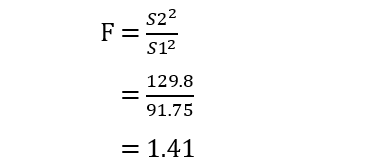### Introduction

F-test is a statistical hypothesis test that compares the variances of two samples so as to test the hypothesis that the samples have been taken from populations with different variances. Usually, F-test is used when there is only a possibility of a two-tailed test when the hypothesis is built. The most prominent test is the test of equality of two population variances. F-test plays an important role in the concept of Analysis of Variance (ANOVA).

### Algorithm

In order to find the test for equality of two population variances, the following steps are to be followed:

1. Initialize the null hypothesis.
2. Initialize the alternative hypothesis.
3. Find the mean and variance of both the population.
4.  Using the F-test formula, calculate the F-value.
5. Find out the degrees of freedom and then the equivalent F-value from statistical tables.
6. Infer whether the null hypothesis is accepted or rejected at a particular level of significance.

The formula for finding the value of F is as follows:Here:

• n1 – number of items in the first population.
• n2 – number of items in the second population.
• s1 – sample variance of the first population.
• s2 – sample variance of the second population.

### Implementation

Let us take an example of the time taken by two employees to complete a particular job using different methods as follows:We can find out whether there is any significant difference between these methods at 5% level of significance using F-test.

1. Null hypothesis H0: The variance of both methods are equal.

2. Alternative Hypothesis H1: The variance of both methods are not equal.

3. We can use the table below to find mean and variance:Note: The value of F is always greater than 1. Therefore, the numerator must always be the larger value.

4. The value of F is:5. Degrees of Freedom can be found by subtracting the total number of samples by 1. Here, ndf = 10, 8. At 5% level of significance, the table value of F is 4.301.

6. Since the table value of F is less than the calculated F, we accept the null hypothesis. Therefore, it can be concluded that there is no significant difference between the two methods in the given example.

Let us write a code for implementing the above problem using the S2 IDE for Kotlin. We create a function that takes two arrays as input and returns the f-value as the output.

				
fun f_test(arr1: DoubleArray?, arr2: DoubleArray?): Double
{
val n1 = arr1?.size
val n2 = arr2?.size
var sumx = 0.0
var sx = 0.0
var sumy = 0.0
var sy = 0.0
for (i in arr1!!)
{
sumx += i
}
val x = sumx / n1!!
for (i in arr2!!)
{
sumy += i
}
val y = sumy / n2!!
for (i in arr1) {
sx += Math.pow(i - x, 2.0)
}
sx = Math.sqrt(sx / n1)
for (i in arr2) {
sy += Math.pow(i - y, 2.0)
}
sy = Math.sqrt(sy / n2)
val S1 = sx * sx * n1 / (n1 - 1)
val S2 = sy * sy * n2 / (n2 - 1)
var f = if (S1 &gt; S2)
{
S1 / S2
}
else
{
S2 / S1
}
return f
}



				
val arr1 = doubleArrayOf(66.0, 67.0, 75.0, 76.0, 82.0, 84.0, 88.0, 90.0, 92.0)
val arr2 = doubleArrayOf(64.0, 66.0, 74.0, 78.0, 82.0, 85.0, 87.0, 92.0, 93.0, 95.0, 97.0)
val f = f_test(arr1, arr2)
System.out.println("F value: \$f")
val table_f = 4.301
if (f &lt; table_f)
{
println(&quot;Null hypothesis is accepted.\n\tThere is no significant difference between the variances of the two populations.&quot;)
}
else
{
println(&quot;Null hypothesis is rejected.\n\tSignificant difference is there between the variances of the two proprtions.&quot;)
}



Output:

				
F value: 1.4147138964577657
Null hypothesis is accepted:
There is no significant difference between the variances of the two populations.